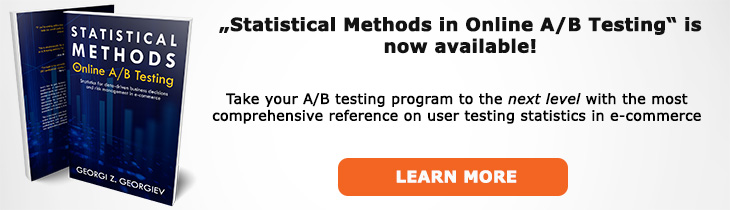# What does "Efficient Estimator" mean?

Definition of Efficient Estimator in the context of A/B testing (online controlled experiments).

## What is an Efficient Estimator?

An efficient estimator is the "best possible" or "optimal" estimator of a parameter of interest. The definition of "best possible" depends on one's choice of a loss function which quantifies the relative degree of undesirability of estimation errors of different magnitudes. When one compares between a given procedure and a notional "best possible" procedure the efficiency can be expressed as relative finite-sample or asymptotic efficiency (a ratio). The relevance to A/B testing is that the more efficient the estimator, the smaller sample size one requires for an A/B test.

When defined asymptotically an estimator is fully efficient if its variance achieves the Rao-Cramér lower bound. Since in many cases the lower bound in the Rao–Cramér inequality cannot be attained, an efficient estimator in statistics is frequently chosen based on having minimal variance in the class of all unbiased estimator of the parameter. Thus optimality in practice is defined using the variance or mean square error (MSE, thus minimum MSE estimator). In other words, the optimal estimator deviates as little as possible from the true value (θ*) one is trying to estimate.

If an efficient estimator exists it is also a sufficient estimator and can be obtained by the maximum-likelihood method (see Maximum Likelihood Estimate).

Like this glossary entry? For an in-depth and comprehensive reading on A/B testing stats, check out the book "Statistical Methods in Online A/B Testing" by the author of this glossary, Georgi Georgiev.## Glossary Index by Letter

Select a letter to see all A/B testing terms starting with that letter or visit the Glossary homepage to see all.